## Lifetime and Line Width

Now we have computed the lifetime of a state. For some atomic, nuclear, or particle states, this lifetime can be very short. We know that energy conservation can be violated for short times according to the uncertainty principleThis means that a unstable state can have an energy width on the order ofWe may be more quantitative. If the probability to be in the initial state is proportional to, then we have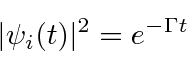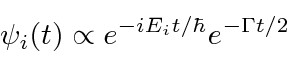We may take the Fourier transform of this time function to the the amplitude as a function of frequency.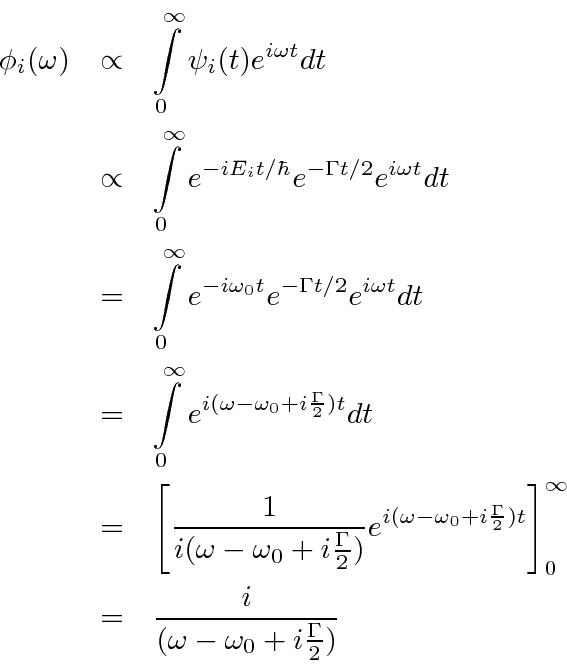We may square this to get the probability or intensity as a function of(and hence.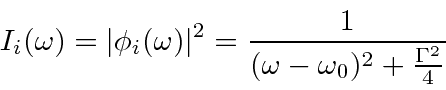This gives the energy distribution of an unstable state. It is called the Breit-Wigner line shape. It can be characterized by its Full Width at Half Maximum (FWHM) of.

The Breit-Wigner will be the observed line shape as long as the density of final states is nearly constant over the width of the line.

Asthis line shape approaches a delta function,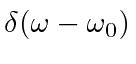.

For the 2p to 1s transition in hydrogen, we've calculated a decay rate ofper second. We can compute the FWHM of the width of the photon line.Since the energy of the photon is about 10 eV, the width is aboutof the photon energy. Its narrow but not enough for example make an atomic clock. Weaker transitions, like those from E2 or M1 will be relatively narrower, allowing use in precision systems.

Subsections
Jim Branson 2013-04-22